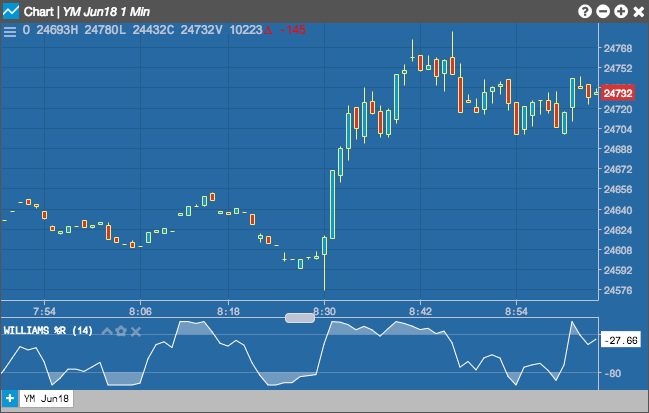Charts

# Williams % R (WillR)

The %R indicator was developed by Larry Williams and introduced in his 1979 book How I Made \$1,000,000 Trading Commodities Last Year. Williams %R is similar to a stochastic oscillator, as it normalizes the price as a percentage between 0 and 100. It is basically an inverted version of the 'Raw %K' value of a Fast Stochastic.

Selecting the Williams %R indicator allows you to set the Period (as number of minutes) and the color of the result.## Configuration Options• Period: Number of bars to use in the calculations.
• Color Selectors: Colors to use for graph elements.
• Over Zones Enabled: Whether to shade the area between the plot and the horizontal overbought and oversold levels.
• Over Bought: Overbought quantity
• Over Sold: Oversold quantity
• Display Axis Label: Whether to display the most recent value on the Y axis.

## Formula

$\%R = -100 \times \left ( \frac{( Highest\; High - Close)}{(Highest\; High - Lowest\; Low )} \right )$

Where:

• Close = the current closing price
• Highest High = the highest high in the past n periods
• Lowest Low = the lowest low in the past  n periods